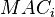# Mean age at birth

(diff) ← Older revision | Latest revision (diff) | Newer revision → (diff)

## Definition

### For all births

The mean age at birth (MAB) or mean age of childbearing (MAC) in a given population over a given time interval is the arithmetic mean of the ages of mothers giving live births at the time of the live birth, for all live births that occur within that time interval.

### For births at a given birth order

Suppose$i$ is a positive integer. The mean age of$i^{th}$ childbearing, denoted$MAC_i$, for a given population in a given time interval is the arithmetic mean of the ages of mothers giving live births at the time of the live birth, for all live births that occur within the time interval at birth order$i$.

## Related cohort notion

For the corresponding cohort fertility notion, see cohort mean age at birth.